# IB DP Physics: HL复习笔记10.1.1 Gravitational & Electrostatic Fields

### Gravitational & Electrostatic Fields

• A field can be defined as

A region in which an object will experience a force, such as gravitational or electrostatic, at a distance

• A gravitational field can be defined as:

The gravitational force per unit mass exerted on a point mass

• An electrostatic field can be defined as:

The electric force per unit charge exerted on a small positive test charge

•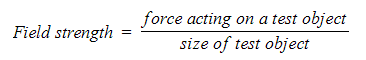Electric field strength, E, and gravitational field strength, g, therefore, have very similar equations
• Despite a few differences, they are analogous to one another in many ways
• In both cases, the nature of the test object is as follows:
• Gravitational fields: small mass, m
• Electrostatic fields: small positive charge, q

#### Uniform Fields

• A gravitational field is a region of space in which objects with mass will experience a force
• The gravitational field strength can be calculated using the equation:
•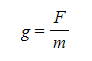Where:
• g = gravitational field strength (N kg-1)
• F = gravitational force on the mass (N)
• m = mass (kg)
• The direction of the gravitational field is always directed towards the centre of the mass
• Gravitational forces are always attractive and cannot be repulsive
• An electric field is a region of space in which an electric charge will experience a force
• The electric field strength can be calculated using the equation:
•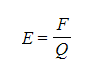Where:
• E = electric field strength (N C-1)
• F = electrostatic force on the charge (N)
• Q = Charge (C)
• It is important to use a positive test charge in this definition, as this determines the direction of the electric field
• The electric field strength is a vector quantity, it is always directed:
• Away from a positive charge
• Towards a negative charge
• Opposite charges (positive and negative) attract each other
• Conversely, like charges (positive-positive or negative-negative) repel each other
• The magnitude of the electric field strength in a uniform field between two charged parallel plates is defined as: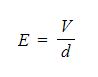• Where:
• E = electric field strength (V m-1)
• V = potential difference between the plates (V)
• d = separation between the plates (m)
• The electric field strength is now defined by the units V m–1
• Therefore, the units V m–1 are equivalent to the units N C–1
• The equation shows:
• The greater the voltage (potential difference) between the plates, the stronger the field
• The greater the separation between the plates, the weaker the field
• This equation cannot be used to find the electric field strength around a point charge (since this would be a radial field)
• The direction of the electric field is from the plate connected to the positive terminal of the cell to the plate connected to the negative terminal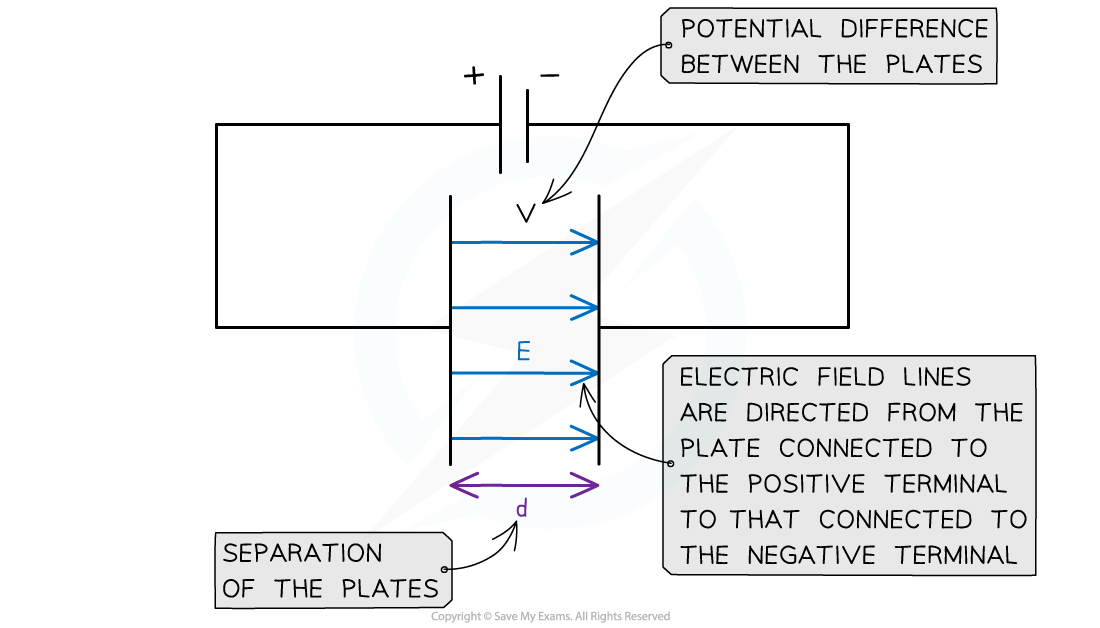The E field strength between two charged parallel plates is the ratio of the potential difference and separation of the plates

• Note: if one of the parallel plates is earthed, it has a voltage of 0 V

• A point charge or mass produces a radial field
• A charged sphere also acts as a point charge
• A spherical mass also acts as a point mass
• Radial fields always have an inverse square law relationship with distance
• This means the field strength decreases by a factor of four when the distance r is doubled
• The gravitational force FG between two masses is defined by:Gravitational vs Electrostatic Forces
• The similarities and differences between gravitational and electrostatic forces are listed in the table below:

Comparing G and E Fields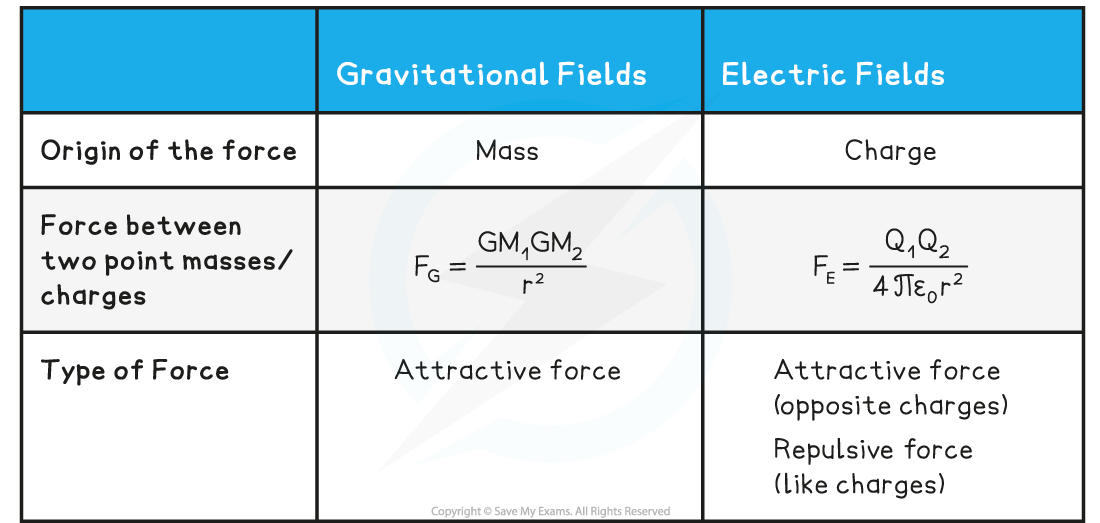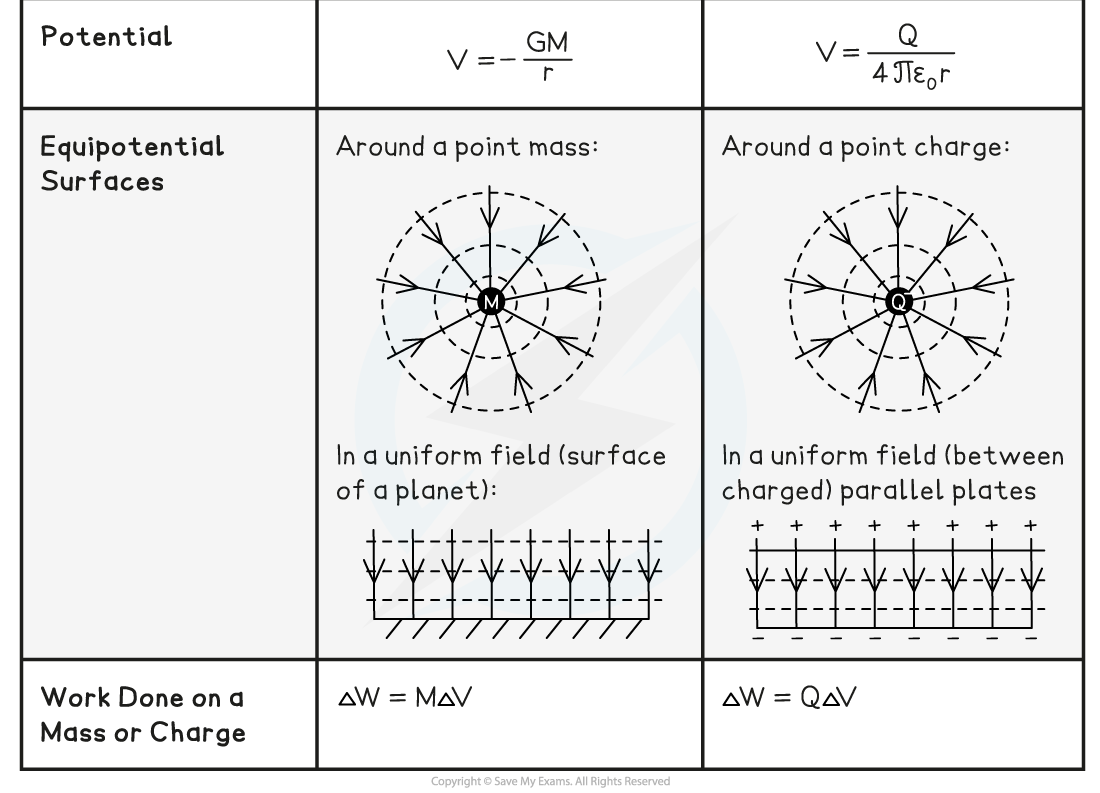• The key similarities are:
• The magnitude of the gravitational and electrostatic force between two point masses or charges are inverse square law relationships
• The field lines around a point mass and negative point charge are identical
• The field lines in a uniform gravitational and electric field are identical
• The gravitational field strength and electric field strength both have a 1 / r2 relationship in a radial field
• The gravitational potential and electric potential both have a 1 / r relationship
• Equipotential surfaces for both gravitational and electric fields are spherical around a point mass or charge and equally spaced parallel lines in uniform fields
• The work done in each field is either the product of the mass and change in potential or charge and change in potential
• The key differences are:
• The gravitational force acts on particles with mass whilst the electrostatic force acts on particles with charge
• The gravitational force is always attractive whilst the electrostatic force can be attractive or repulsive
• The gravitational potential is always negative whilst the electric potential can be either negative or positive

#### Worked Example

Two parallel metal plates are separated by 3.5 cm and have a potential difference of 7.9 kV.

Calculate the electric force acting on a stationary charged particle between the plates that has a charge of 2.6 × 10-15 C.

Step 1: Write down the known values

• Potential difference, V = 7.9 kV = 7.9 × 103 V
• Distance between plates, d = 3.5 cm = 3.5 × 10-2 m
• Charge, Q = 2.6 × 10-15 C

Step 2: Calculate the electric field strength between the parallel plates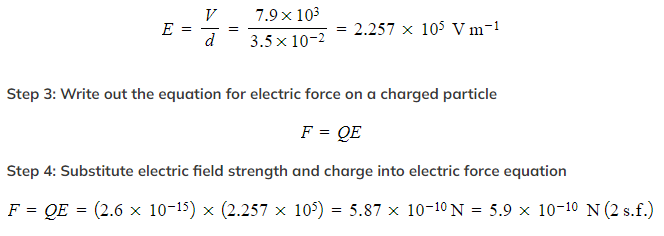Exam Tip

Remember to use the correct equation depending on whether there is a uniform or radial field.

For electric fields:

• Uniform fields: parallel plates / capacitors
• Radial fields: around point charges

For gravitational fields:

• Uniform fields: near the Earth's surface
• Radial fields: around masses (e.g. planets and moons)

You should be able to tell the type of field from the field lines. Uniform fields have equally spaced, parallel field lines.